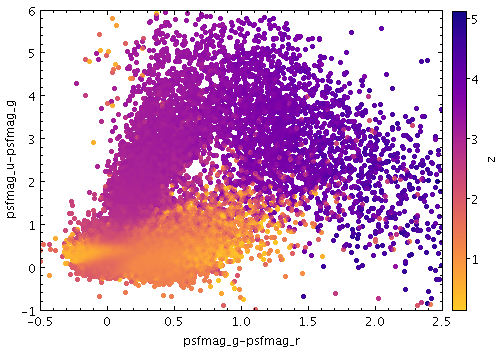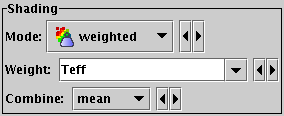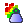Next Previous Up Contents
Next: Colour Maps
Previous: Aux Mode

#### A.4.6.7 Weighted ModeWeighted mode selection

The Weighted shading mode () paints markers using colours indicating density at each pixel like the Density mode, but with an optional weighting coordinate. You can configure how the weighted coordinates are combined at each pixel to give the final weighted result. Depending on the configuration and actual point density, this can behave in some ways like Aux mode and in some ways like Density mode, but allows you to do things that are not possible in either.

The shading is done using the shared colour map. This colour map is used by all currently visible Weighted, Aux, Grid and SkyDensity layers. When at least one such layer is being plotted, the Aux Axis control is visible in the control panel, which allows you to configure the colour map, range, ramp display etc.

The options are:

Weight
The weight value applied to each plotted point. Fill this in with a column name or expression from the table just like for a positional coordinate. The exact way this quantity is used depends on the setting of the Combine control below. If it's left blank, the weighting is considered to be unity (all values are 1); this makes sense for some combination types (e.g. sum) but not others (e.g. mean).
Combine
Determines how the weight values associated with markers plotted covering a given screen pixel are combined to produce the numeric value used for that pixel's colour.

The following options (some are more useful than others) are currently available:

• `sum`: the sum of all weights
• `mean`: the mean of all weights
• `median`: the median of all weights (may be slow)
• `q1`: the first quartile of all weights (may be slow)
• `q3`: the third quartile of all weights (may be slow)
• `min`: the minimum weight
• `max`: the maximum weight
• `stdev`: the sample standard deviation of all weights
• `count`: the number of points plotted (weight value is ignored, this is like Density mode)
• `hit`: one if any point is plotted, blank otherwise (weight value is ignored, this is like Flat mode)

Exporting: When exported to vector formats, the output is automatically forced to a bitmap for Density-mode layers. In the case of PostScript, this completely obscures any previous layers.

Next Previous Up Contents
Next: Colour Maps